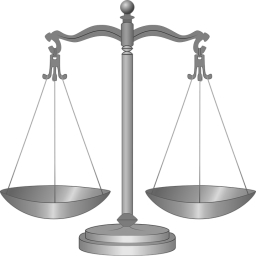# Matemakak 9432

The cookbook by Matěj Matemakak says:
The greatest common divisor of flour weight and sugar weight is 15, the greatest common divisor of sugar weight and lemon peel weight is 6, the product of sugar weight and lemon peel weight is 1800, and the smallest common multiple of flour weight and sugar weight is 3150.
What are flour, sugar, and lemon peel weights in a lemon pie recipe?

m =  315 g
c =  150 g
k =  12 g

### Step-by-step explanation:Did you find an error or inaccuracy? Feel free to write us. Thank you!

Tips for related online calculators
Do you want to calculate the least common multiple of two or more numbers?
Do you want to calculate the greatest common divisor of two or more numbers?
Do you have a system of equations and looking for calculator system of linear equations?